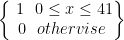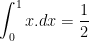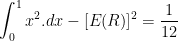# Properties of Random Numbers in Simulation

A sequence of random numbers R1, R2, RR3......... must have two important properties.

i) Uniformity i.e. they are equally probable everywhere.

ii) Independence, i.e. the current value of a random variable has no relation with previous values.

Each Random Number Ri is an independent sample drawn from a continuous uniform distribution between zero and one.

• pdf f(x) =• expectation E(R) =• variance V(R) =Some consequences of the uniformity and independence properties.

i) If the interval(0,1) is divided into n sub-intervals of equal length, the expected number of observations in each interval is N/n where N is the total number of observations. Note that N has to be sufficiently large to show this trend.

ii) The probability of observing a value in a particular interval is independent of the previous values drawn.